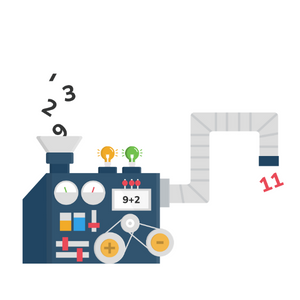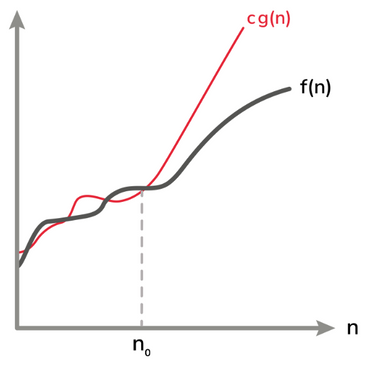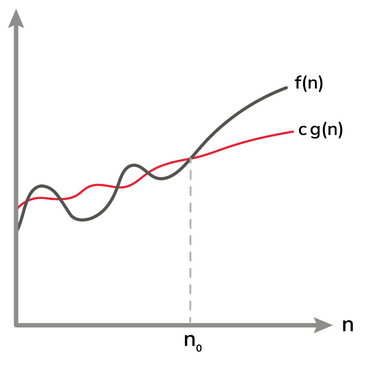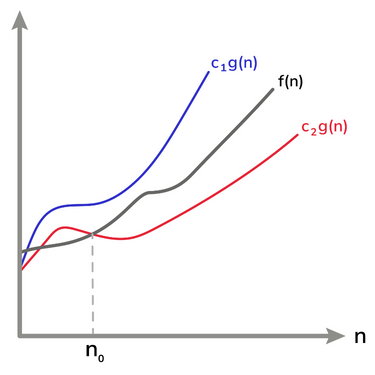Home/Blog/Programming/Asymptotic runtime complexity: How to gauge algorithm efficiency

# Asymptotic runtime complexity: How to gauge algorithm efficiency

Aug 22, 2022 - 17 min readAlgorithms are behind every computer program. To solve the same problem, usually, several algorithms can be designed. Thus, finding the best algorithm is the key to saving time and money and providing the best customer service.

Some algorithms are so inefficient (slow) that they cannot be used in practice. In such a case designing a new, more efficient algorithm can drastically change the landscape. For example, the Fourier transformation algorithm was slow and not usable. Thus, the invention of the Fast Fourier transform made a large variety of applications possible, including digital recording, image processing, pitch correction, etc.

This blog answers a fundamental question: how to calculate an algorithm’s efficiency. By answering it, we can compare algorithms to find the most suitable algorithm for a given task. This is an essential skill for a programmer to create a fast, memory-efficient, and responsive program that performs predictability under a known bound in all circumstances. Thus, any customer-centric enterprise will always use algorithms with known efficiency and memory bounds that are also proven to be correct. This blog will also teach you about the worst-case and the best-case time complexities of an algorithm and how to find them. We will also learn about famous asymptotic notations: Big O, Big Omega, and Big Theta.

The blog uses mathematical notations and formulas which might look tedious to some readers. Worry not! We have also described those notations in simple English, with graphical illustrations, and given examples for clarity.

Let the fun begin!

#### Get hands-on with algorithms today.

Try one of our 300+ courses and learning paths: Data Structures and Algorithms in Python.

## What is an algorithm

An algorithm refers to a sequence of steps that solves a given problem. To that end, an algorithm takes some input(s) and produces desired output(s). For example, below is the input and the corresponding output of a typical sorting algorithm:

Input: An array of numbers $[a_1, a_2, \cdots, a_n]$

Output: A sorted array of numbers $[\hat{a_1}, \hat{a_2},\cdots, \hat{a_n}]$, where $\hat{a_i} \leq \hat{a}_{i+1}, \forall i$

An algorithm is considered correct if and only if, on all the possible inputs, it produces the desired output(s). For instance, a sorting algorithm that always sorts an array of numbers successfully for all the possible inputs will be considered correct.We usually write an algorithm in pseudocode. Pseudocode is a mixture of English and a programming-language-independent syntax that is easy to understand by a layperson. Below is an algorithm’s pseudocode to find the minimum number from an unsorted array of numbers.

// function takes an unsorted array as input and returns minimum value find_min (A[a_1, a_2, ..., a_n])  // Assume that the first element is the minimum  // In pseudocode we assume first index as 1  min = A  // for loop from the second index of array to its last element  for j=2 to n      // if any element is less than minimum then make it minimum      if A[j] < min          min = A[j]  // returns the minimum value  return min
Pseudocode of finding minimum from a given array

## Independent time complexity

When we implement an algorithm in a programming language (such as Java or C), it becomes a program and can be executed on various hardware platforms. Different algorithms designed to solve the same problem usually differ widely in their time complexities. This difference may change drastically or be skewed when these algorithms are implemented in different programming languages and are executed on different hardware platforms. For instance, in general, an algorithm implemented in Assembly language would execute faster than its Python implementation. Similarly, an algorithm (say Algo-n) running on a supercomputer will be faster than another algorithm (Algo-m) running on an old resource-constrained PC. This might be true even when Algo-m is theoretically more efficient than Algo-n.

We cannot fairly compare algorithms’ efficiency by executing their implementations and recording their runtime.

Therefore, computer scientists and programmers are interested in knowing an algorithm’s time complexity independent of its implementation’s language and execution time on any hardware platform.

#include <stdio.h>// function takes an unsorted array as input and returns minimum value int find_min (int A[], int length) {  // Assume that the first element is the minimum  // in C array index starts at 0  int min = A;  // for loop from the second index of array to its last element  for (int j=1; j < length; j++) {      // if any element is less than minimum then make it minimum      if (A[j] < min)          min = A[j];  }  // returns the minimum value  return min;  }// The main functionint main() {  int A[] = {2, 7, 9, -5, 4, 5, 3, 1};  printf("minimum = %d", find_min(A, 8));  return 0;}
Implementation of find_min algorithm in C language

### Time complexity as function of input’s size

Most algorithms’ time complexity will increase as their input size increases. For instance, if the input to the find_min algorithm is an array of size 10, it will run faster as compared to when its input is an array containing 1 million elements.

If Algo-1 is faster on smaller inputs than Algo-2 but slower on large inputs, will the Algo-1 be considered more efficient?

In computer science, we are interested in the time complexity of an algorithm as a function of the size of its input. That is, how the time complexity of an algorithm grows with respect to the increase in its input size. Thus, an algorithm (in this case Algo-2) that is generally more efficient on the larger input sizes is considered superior.

### Calculating time complexity

We aim to measure an algorithm’s time complexity independent of its implementation and any hardware platform. To that end, we assume that each pseudocode instruction will take a constant amount of time. In particular, $i^{th}$ pseudocode instruction will take $c_i$ time to execute, where $c_i$ is a positive integer. We will carefully calculate how many times each instruction will execute. Then we calculate the total time taken by each instruction as:

Total time of Instruction-i = constant time taken by it, $c_i$ $\times$ number of times it will be executed

Finally, we add the total time taken by all the instructions of the pseudocode, to calculate the time taken by our algorithm.

Let’s make it crystal clear by calculating the time of our find_min pseudocode given earlier.

Code Cost of instruction Number of times executed
find_min (A[a_1, a_2, ..., a_n]) $c_1$ 1
min = A $c_2$ 1
for j=2 to n $c_3$ $n$
if A[j] < min $c_4$ $n-1$
min = A[j] $c_5$ $k$
return min $c_6$ 1

Thus, the total time taken by our find_min algorithm on given input of size $n$ is:

\begin{align*} T(n) &= c_1+c_2+nc_3+(n-1)c_4+k c_5+c_6 \\ &= (c_1+c_2-c_4+c_6)+n(c_3+c_4)+k c_5\\ &= a+nb+kc \end{align*}

In the equation above, $a=c_1+c_2-c_4+c_6$, $b=c_3+c_4$, and $c=c_5$. Furthermore, the value of $k$ depends on the kind of input. We can understand the concept of the worst case and the best case behavior of the algorithm with the possible values the constant $k$ may take.

The best case occurs when the minimum integer will be the first element of the array. Hence, the if-condition will never be true. Thus, the value of $k$ in the best case will be zero, and $T(n)=a+nb$. Whereas in the worst case the input array will be sorted in descending order. Hence, the if-condition will always be true, making the value of $k$ in the worst case $n$, $T(n)=a+nb+nc$.

## Asymptotic time complexity

In the last section, we learned to find the running time of iterative algorithms. However, we may have two algorithms with different running times:

Algo-1 Alog-2
$T_1(n)=an^2+bn+d$ $T_2(n)=en^2+fn$

We cannot say which one is better without having to calculate the exact values of constants $a$, $b$, $d$, $e$, and $f$. However, these constant values will not be independent of the implementation language and hardware platform. Furthermore, finding their exact values will be too cumbersome. Thus, we resort to asymptotic time complexity. In asymptotic time complexity, our focus is on the order of growth of the running time corresponding to the increase of the input size. Big O, Big Theta, and Big Omega are three key notations that describe asymptotic time complexity.

### Big O: The upper bound

Given that a running time of an algorithm is $T(n)=f(n)$, where $n$ is the size of the input, we say that function f is big O of a function g, written as:

$f(n) = O(g(n))\text{,}$

if there exist positive constants $c, n_0 \in \R$ such that

\begin{align*} f(n) \le c g(n),\ \ \forall n \ge n_0 \end{align*}\text{.}

In simple words, the above equation implies that when the size of input $n \ge n_0$, the time of the algorithm $f(n)$ will be upper bounded by $c g(n)$. That is, $f(n)$ will be equal to or less than $c g(n)$ on all the input’s sizes $n \ge n_0$. This is depicted by the following graphical illustration.A common mistake is referring to the Big O time complexity as the worst case of an algorithm. On the contrary, Big O refers to the upper bound, which could be for the best case, the worst case, or the average case.

If $f(n) = O(n^i)$, for any integer $i$, then by the above definition, the following statement will always be true:

$\begin{equation*} f(n) = O(n^j), \forall j \ge i \end{equation*}$

### Big Omega: The lower bound

Given that a running time of an algorithm is $T(n)=f(n)$, where $n$ is the size of the input, we say that function f is Big Omega of a function g, written as:

$f(n) = \Omega(g(n))\text{,}$

when there exist positive constants $c, n_0 \in \R$, such that

$f(n) \ge c g(n),\ \ \forall n \ge n_0\text{.}$

In simple words, the above equation implies that when the size of input $n \ge n_0$, the time of the algorithm $f(n)$ will be lower bounded by $c g(n)$. That is, $f(n)$ will be equal to or greater than $c g(n)$ on all the input’s sizes $n \ge n_0$, as depicted by the following illustration.Some people incorrectly refer to Big Omega as an algorithm’s best case time complexity. On the contrary, Big Omega refers to the lower bound, which could be for the best case, the worst case, or the average case.

If $f(n) = \Omega(n^i)$, for any integer $i$, then by the above definition, the following statement will always be true:

$\begin{equation*} f(n) = \Omega(n^j), \forall j \le i \end{equation*}$

### Big Theta: The tight bound

Big Theta is the most useful asymptotic notation and should be used in place of Big O and Big Omega whenever possible. It is because it represents the tight bound of running time and provides much more information about an algorithm’s complexity than its counterparts.

Given that a running time of an algorithm is $T(n)=f(n)$, where $n$ is the size of the input, we say that function f is Big Theta of a function g, written as:

$f(n) = \Theta(g(n))\text{,}$

if there exist positive constants $c_1, c_2, n_0 \in \R$ such that

$c_2 g(n) \le f(n) \le c_1 g(n),\ \ \forall n \ge n_0 \text{.}$

In simple words, the above equation implies that $f(n)=\Theta(g(n))$ as well as $f(n)=O(g(n)$ for input size $n \ge n_0$, with constants $c_1$ and $c_2$, respectively. In other words, $f(n)$ will be equal to or less than $c_1(g(n))$ as well as equal to or greater than $c_2(g(n))$ on all the input’s size $n \ge n_0$. This is depicted by the following graphical illustration.## Example: Insertion sort

Let’s go through an example to make all the concepts clear. Insertion sort is a famous sorting algorithm that is similar to the typical sorting of cards.Insertion sort is similar to sorting cards

### Explanation of algorithm

In Insertion sort, we assume that our input array is divided into two parts: sorted elements and unsorted elements. Initially, the sorted array has only a single element, whereas the unsorted array has the rest of the elements. We remove an element from the unsorted part of the array and find the place to insert it at the correct location in the already sorted array. This process continues until the unsorted part becomes empty. The pseudocode of Insertion sort is given below:

//input is an array of integersInsertion_sort(A[a_1, a_2, ..., a_n])  //A[1 .. i-1] is our sorted list.  //A[i .. A.length] is our unsorted list.   //Initally i=2 so sorted list has a single element in it.  for i=2 to n      toInsert = A[i] //toInsert is the element we want to insert at correct location in sorted list        for j=i-1; j > 0 and A[j] > toInsert; j=j-1          A[j+1] = A[j]      // now insert toInsert at its correct location in sorted array      A[j+1] = toInsert
Insertion sort pseudocode

### Insertion sort running time

Code Cost of instruction Number of times executed
Insertion_sort(A[a_1, a_2, ..., a_n]) $c_1$ 1
for i=2 to n $c_2$ n
toInsert = A[i] $c_3$ $n-1$
for j=i-1; j > 0 and A[j] > toInsert; j=j-1 $c_4$ $h(j)$
A[j+1] = A[j] $c_5$ $h(j)-1$
A[j+1] = toInsert $c_6$ $n-1$

According to the calculations presented in the table above, the running time of Insertion sort for an input of size $n$ is:

\begin{align*} T(n) &= c_1+ c_2 n+ c_3 (n-1)+c_4 (h(j))+ c_5 (h(j)-1)+c_6 (n-1)\\ &= (c_2+c_3)n + (c_4+c_5)h(j)+ (c_1-c_3-c_5-c_6)\\ &= an+bh(j)+c \end{align*}

Where, constants $a=c_2+c_3$, $b=c_4+c_5$, and $c=c_1-c_3-c_5-c_6$

### Best-case analysis

In the best case, the condition $A[j] > \text{toInsert}$, is never true. That is, in this case, the input array is already sorted. Thus, in the best case $h(j)=1$. The running time becomes:

$T(n) = an+b+c = an+d$

We claim that $T(n)=\Theta(n)$. To prove that claim, we have to show that $T(n)=O(n)$ and $T(n)=\Omega(n)$. Let’s verify it quickly.

To show that $T(n)=O(n)$, we have to find a positive constant $c_1$ such that $an+d \le c_1n$. One such possibility could be $c_1=|a|+|d|$. For all $n>=1$ the above inequality holds, proving that $T(n)=O(n)$.

To show that $T(n)=\Omega(n)$, we have to find a positive constant $c_2$ such that $an+d \ge c_2n$. One possibility could be $c_2=1$. For all $n>=1$ the above inequality holds, proving that $T(n)=\Omega(n)$.

Thus, proving that the best-case complexity analysis of Insertion sort is $\Theta(n)$.

### Worst-case analysis

In the worst case, the condition $A[j] > \text{toInsert}$, will always be true. In this case, the input array is reversely sorted (descending order). In our pseudocode, the loop (of line number 8) will always run from $j=i-1$ to 0. Therefore, $h(j)=1+2+3+\cdots+n$. We can calculate this sum using the sum of arithmetic series formula, $h(j)=\frac{n}{2}(1+n)=\frac{n}{2}+\frac{n^2}{2}$. Thus,

$T(n) = a\bigg(\frac{n}{2}+\frac{n^2}{2}\bigg)+c+b = an+d$

We claim that according to the above equation, the worst-case running time of Insertion sort is $T(n)=\Theta(n^2)$. We can easily prove it using arguments similar to the best-case proof. However, we are leaving it to the readers.

#include <stdio.h>//input is an array of integersvoid Insertion_sort(int A[], int n) {  //A[1 .. i-1] is our sorted list.  //A[i .. A.length] is our unsorted list.   //Initally i=1 so sorted list has a single element in it.  for (int i=1; i<n; i++) {      int toInsert = A[i]; //toInsert is the element we want to insert at correct location in sorted list        int j=i-1;      for (j=i-1; j >= 0 && A[j] > toInsert; j--) {          A[j+1] = A[j];      }      // now insert toInsert at its correct location in sorted array      A[j+1] = toInsert;   }}int main() {    int A[]={19, 9, 3, 1, 2, 19, 23, 0};    Insertion_sort(A,  8);    printf("\nSorted array is = {");    for (int index=0; index < 8; index++) {        printf("%d", A[index]);        if (index+1 < 8) printf(", ");    }    printf("}\n");    return 0;}
Insertion sort implementation in C language

## Time complexities of sorting algorithms

Let’s conclude this blog by presenting the best- and the worst-case complexity of some of the famous sorting algorithms, given that they take an array of size $n$ as input.

Algorithm Best case Worst case Average case
Insertion sort $\Theta(n)$ $\Theta(n^2)$ $\Theta(n^2)$
Quicksort $\Theta(n\log n)$ $\Theta(n^2)$ $\Theta(n\log n)$
Merge sort $\Theta(n\log n)$ $\Theta(n\log n)$ $\Theta(n\log n)$
Bubble sort $\Theta(n)$ $\Theta(n^2)$ $\Theta(n^2)$
Selection sort $\Theta(n^2)$ $\Theta(n^2)$ $\Theta(n^2)$
Heap sort $\Theta(n\log n)$ $\Theta(n\log n)$ $\Theta(n\log n)$

Test your understanding and learn from mistakes.

1

Given an algorithm’s worst-case time complexity in $\Theta(W(n))$, and its best-case complexity is $\Theta(B(n))$, then its average-case complexity $A(n)$ must be

###### A)

$A(n)=O(B(n))$ and $A(n)=\Omega(W(n))$

###### B)

$A(n)=\Omega(B(n))$ and $A(n)=O(W(n))$

###### C)

$A(n)=\Omega(B(n))$ and $A(n)=\Omega(W(n))$

###### D)

$A(n)=O(B(n))$ and $A(n)=O(W(n))$

Question 1 of 70 attempted

#### Get hands-on with algorithms today.

Try one of our 300+ courses and learning paths: Algorithms for Coding Interviews in Java.

## Increase your algorithm proficiency today

At this point, you should have a pretty good understanding of the importance of calculating an algorithm’s efficiency, how to do so, the reasons for using asymptotic time complexity, and the differences between its key notations.

In case you want to learn about algorithms, and their time complexities, in more detail, then you’ll find a plethora of interactive and exciting courses available at Educative. If you’re relatively new to this subject, consider the course Data Structures and Algorithms in Python, which covers these fundamental computer science concepts using Python, an essential language for developers and data scientists alike. If you know a little Java and are looking to take the next step in your career, we’d suggest checking out the course Algorithms for Coding Interviews in Java.

Happy learning!